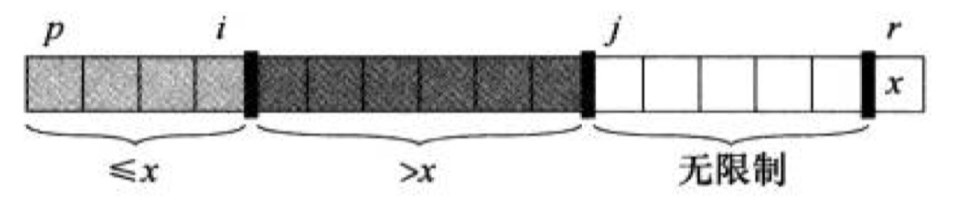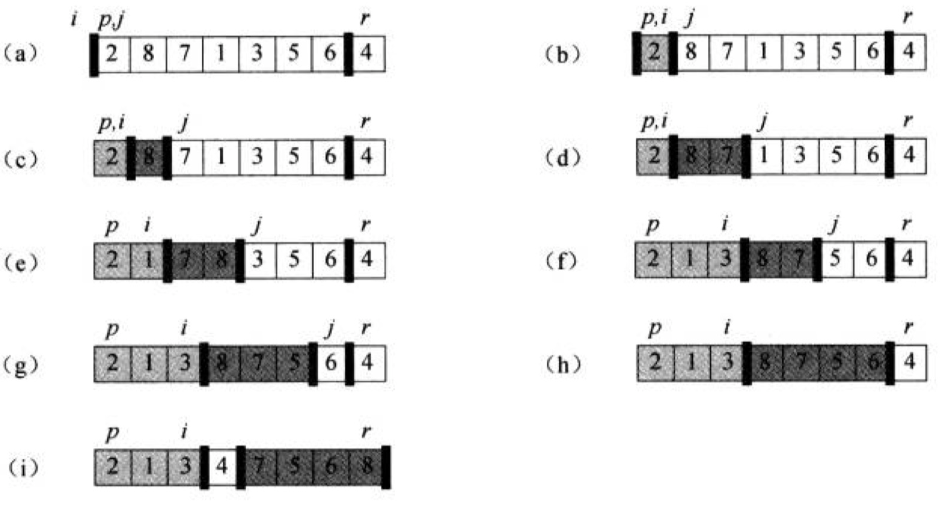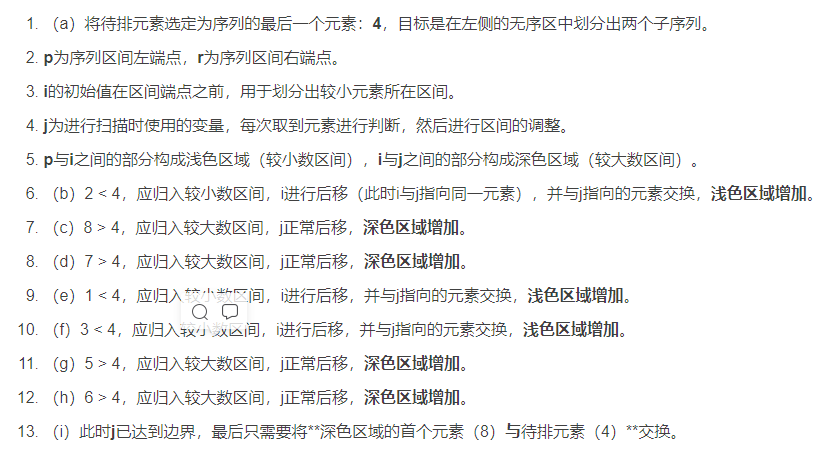+关注继续查看

# 算法概念• 数组
• 队列
• 链表
• 树等等

• 时间复杂度
• 空间复杂度

• 冒泡排序

• 快速排序

# 快速排序

• 输入

n个数的序列，通常直接存放在数组中，可能是任何顺序。

• 输出

• 算法说明

• 算法流程# 伪代码

FUNCTION PARTITION(A,p,r)
x =  A[r]
i = p - 1
for j = p to r - 1
if a[j] < x
i = i + 1
exchange A[i] with A[j]
exchange A[i + 1] with A[r]
return i + 1

PARTITION函数的作用时进行第一趟排序，排好一个元素，并得到两个子序列，返回值就是接下来划分子序列的参照。

FUNCTION QUICKSORT(A,p,r)
if p < r
q = PARTITION(A,p,r)
QUICKSORT(A,p,q-1)
QUICKSORT(A,q+1,r)

QUICKSORT函数进行了递归的操作，将原问题分解为一系列的子问题，每次都在序列上进行划分、调用的操作，每次不断的改变区间的长度，直到不需要再划分为止。

# 算法实践

• 输入数据： 2，8，7，1，3，5，6，4

java源代码

public class QuickSort {

public static void main(String[] args) {
// input data
int[] a = {2,8,7,1,3,5,6,4};
// 调用快速排序，传入初始的左右端点
quickSort(a,0,a.length - 1);
// 查看排序结果
for (int data : a){
System.out.print(data + "\t");
}
}

private static int partition(int[] a,int p,int r){
// 声明待排元素
int x = a[r];
// 初始化较小数区间端点
int i = p - 1;
// 循环结束后，区间已经划定完毕
for(int j = p;j < r;j++){
// 将较小的数向前扔
if(a[j] < x){
i++;
// 交换两个元素
int tmp = a[i];
a[i] = a[j];
a[j] = tmp;
}
}
// 交换待排元素到指定位置
int tmp = a[i + 1];
a[i + 1] = a[r];
a[r] = tmp;
return i + 1;
}

private static void quickSort(int[] a,int p,int r){
// 重要：递归的出口（终止条件）为区间长度小于1
if(p < r){
// 划分后得到已排好元素的位置
int q = partition(a,p,r);
// 根据位置得到较小数列区间
quickSort(a,p,q-1);
// 根据位置得到较大数序列区间
quickSort(a,q + 1,r);
}
}

}

• 执行效果

1 2 3 4 5 6 7 818 033 035 0747 01471 0956 0742 01200 0+关注
122

0